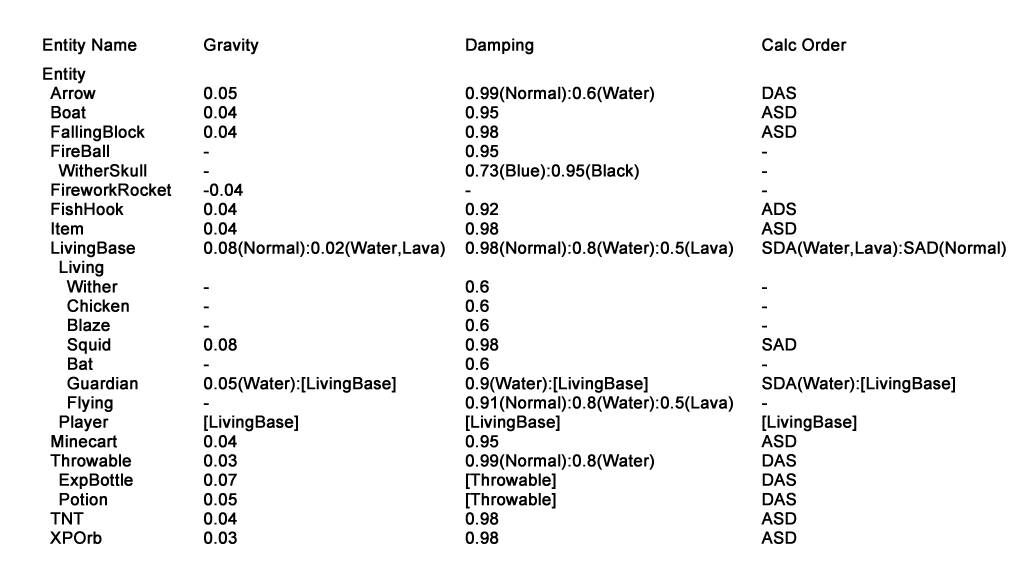#### 【游戏机制】自由落体的运动规律——理论部分

12-19 3,571 views

## 自由落体的运动规律Gravity表示的就是加速作用，单位是m/gametick^2；Damping表示的就是阻尼作用，单位是1/gametick。Calc Order表示的是加速作用和阻尼作用的先后顺序，A表示加速作用，D表示阻尼作用，S表示计算路程。

## 关于自由落体的速度

### 1）加速先于阻尼

v(1)=0······(1)

v(t+1)=[v(t)+a*1](1-f)=[v(t)+a](1-f)······(2)

v(t+1)-v(t)=[v(t)+a](1-f)-v(t)=a-f[a+v(t)]······(3)

v’=a(1/f-1)······(4)

v(t)的递推公式其经过等式变换以后，可以得到下面的形式：

[v(t+1)+L]=[v(t)+L](1-f)······(5)

v(t+1)=[v(t)+L](1-f)-L=[v(t)+L-L/(1-f)](1-f)······(6)

L-L/(1-f)=a······(7)

L=-a(1/f-1)······(8)

[v(t+1)-v’]=[v(t)-v’](1-f)······(9)

v(t)-v’=[v(1)-v’](1-f)^(t-1)······(10)

v(t)=[v(1)-v’](1-f)^(t-1)+v’······(11)

v(t)=v'[1-(1-f)^(t-1)]······(12)

daltav(t)=v'(1-f)^(t-1)······(13)

e=v'(1-f)^(t’-1)······(14)

t’=ln(e/v’)/ln(1-f)+1······(15)

### 2）阻尼先于加速

v(1)=0······(1)

v(t+1)=v(t)(1-f)+a*1=v(t)(1-f)+a······(2)

v’=a/f······(3)

v(t)=v'[1-(1-f)^(t-1)]······(4)

t’=ln(e/v’)/ln(1-f)+1······(5)

## 关于自由落体的下落高度

### 1）ASD模式

ASD模式中，第t个游戏刻内实体的下落高度h'(t)为：

h'(t)=[v(t)+a]*1=a/f-v'(1-f)^(t-1)······(1)

h(t)=[h'(1)+h'(2)+……+h'(t)]/(1-f)=[v(1)+v(2)+……+v(t)]+at=v'[t-S(t)]+at······(2)

S(t)=1+(1-f)+(1-f)^2+……+(1-f)^(t-1)······(3)

S(t)=[1-(1-f)^t]/f······(4)

h(t)={at-v'[1-(1-f)^t]}/f······(5)

h'(t)=v'[1-(1-f)^t]······(1)

h(t)=v'{t-(1-f)[1-(1-f)^t]/f}······(2)

h'(t)=v'[1-(1-f)^(t-1)]······(1)

h(t)=v'{t-[1-(1-f)^t]/f}······(2)

## 总结

A：一个游戏刻内，对实体加速的计算

D：一个游戏刻内，对实体阻尼的计算

S：一个游戏刻内，对实体下落高度的计算

t：时间，单位为gametick，表示自由落体开始后的游戏刻数量

a：加速度，单位为m/gametick^2，表示的是每个游戏刻末与初的速度净增加量

f：阻尼，单位为1/gametick，表示的是每个游戏刻末与初的速度比值

v’：最终下落速度，单位为m/gametick，表示的是经过足够长的时间后，实体匀速下落时的速度

v(t)：下落速度，单位为m/gametick，表示自由落体开始后第t个游戏刻初的下落速度，且v(1)=0

h'(t)：单位时间的下落高度，单位为m，表示自由落体开始后第t个游戏刻内的下落高度

h(t)：下落总高度，单位为m，表示自由落体开始t个游戏刻后的下落高度

e：高度测量精度，其值为10^(-5)m，即0.00001m

t’：加速时间，单位为gametick，表示实体加速至最终下落速度所需要的时间。

v(1)=0

v(t+1)=[v(t)+a*1](1-f)=[v(t)+a](1-f)

v’=a(1/f-1)······(4)

v(t)=v'[1-(1-f)^(t-1)]

t’=ln(e/v’)/ln(1-f)+1

v(1)=0

v(t+1)=v(t)(1-f)+a*1=v(t)(1-f)+a

v’=a/f

v(t)=v'[1-(1-f)^(t-1)]

t’=ln(e/v’)/ln(1-f)+1

h'(t)=[v(t)+a]*1=a/f-v'(1-f)^(t-1)

h(t)={at-v'[1-(1-f)^t]}/f

h'(t)=v'[1-(1-f)^t]

h(t)=v'{t-(1-f)[1-(1-f)^t]/f}

h'(t)=v'[1-(1-f)^(t-1)]

h(t)=v'{t-[1-(1-f)^t]/f}

Dim White（百度id：滚大幕）提供的redstone_player皮肤及自由落体机制的讨论。

gp（百度id：Gamepiaynmo）提供的关于游戏代码中实体自由落体的机制和相关参数。

【游戏机制】刷怪机制的可视化

【15w43a快照】1.9独立的CB系统及其新应用